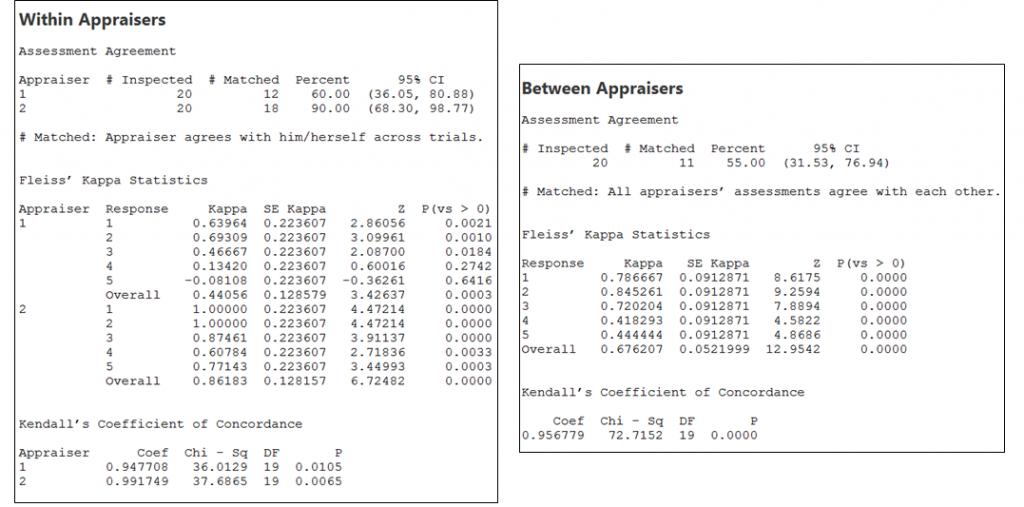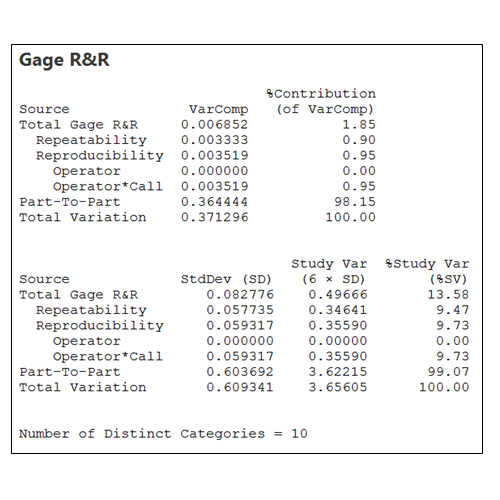## Lean Six Sigma Black Belt Sample Paper: Measure

1. Which of the following statements is NOT true about Attribute Data?
2. Select the logical sequence of various steps followed in the Measure phase of a DMAIC project?
3.  VOC - Room is too hot. If the temperature is more than 24, it will be hot and less than 18, it will be too cold. Why can we not keep it at 21? What is the LSL, USL and the Target?
4. Which of the following statement is correct while considering Attribute Agreement Analysis?
5. What is the pull down menu for Attribute Agreement Analysis in Minitab?
6. In context of Measurement System Analysis, which of the following statement is NOT true?
7.  In an inspection of 200 projectors, 5 defects are found in 3 projectors and remaining projectors had no defects. What is the DPU and Zlt?
8. Which of the following statement is NOT true about the Process Capability and Performance Indices?
9. A dye manufactures 10mm diameter bolts. If the bolt dimensions are more than +/- 0.01mm, they do not fit. The standard deviation measured is 0.05 and the mean is 10mm. What is the Sigma Level (Zlt)?
10. Considering the various Sigma Level calculations, what will be the most plausible ascending order of their values?
11. A quality team does visual inspection for scratches on the phone screen. If there is a scratch, the phone is deemed as defective. What is the best method to be used to calculate current performance level?
12. What is the Rolled Throughput Yield for the following process – 100 candidates apply for a job, out of which 75 clear the written test. Out of the 75, 50 clear the interview, all 50 are given the job offer. 35 candidates join.
13. Which of the following elements describe Operational Definition?

i) It ensures common, clear and consistent understanding of a measure

ii) It provides an air tight definition of a variable

iii) It translates general understanding of a variable to specific

iv) It helps impress the stakeholders
14. Interpret the outcome for Attribute Agreement Analysis performed on Ordinal Data Set. Assume that there is no standard score.15. Interpret the results for Gage R&R from the outcome on the sessions window:16. Using the below information, what is the Sigma Level to be communicated to the customer?
USL = 24
LSL = 18
Mean = 21
Standard Deviation = 2.0
17. Which of the following statement that is NOT true for short term and long term performance?
18. 500 projectors were inspected for 5 characteristics each. 150 defects were observed. What is the estimated short term sigma level (Zst)?
19. If the average yield of a process is 95%, what is the the long term Z value?
20. What does Cpk=0 imply?
21. What will be the estimated sigma level for a process which has Specification Width twice as much as the Process Width?
22. Which of the following statement about Non-Normal data is true?
23. Which of the following statements is NOT true about Measurement System Analysis?
24. Which of the following is NOT an objective of the Measure phase?
25. Complete the following statement
Attribute Agreement Analysis is ____________
26. Which of the following statement is NOT true?
27. In order to basket each ball, the player’s throw must be _______________
28. If a weighing scale shows correct weight between the 0-30kgs range, but shows an error of 5kgs in the 30-100kg range, what kind of Gage Error is it?
29. If all appraisers agree with each other but do not agree with the standard, what is the possible action one can take?
30. Instead of using the approximation of 1.5 shift, Z shift can actually be computed for which of the following type of data?
31. If Pp=1.5 and Ppk=0.5, which of the following statement is true?
32.  If the data collection is automated in an industry (say in a contact center), which tool will you use to validate the measurement system?
33. Which of the following is NOT a deliverable of Measure phase?
34. Which of the following will use Crossed Design for Continuous Gage R&R Assessment?
35. Which of the following statement is NOT true?
36. The average turnaround time for resolving a problem ticket is 10 days and standard deviation is 1 day. What is the probability that we will be able to resolve a problem within 11 days? (Assume data follows normal distribution)
37.  For ordinal data, which of the following will indicate the degree of alignment between different operators?